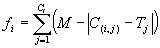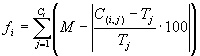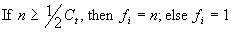GEP Book

Home
News
Author
Q&A
Tutorials
GEP Biblio
Contacts

Visit Gepsoft

 C. FERREIRA Complex Systems, 13 (2): 87-129, 2001

Gene Expression Programming: A New Adaptive Algorithm for Solving Problems

Fitness Functions

One important application of GEP is symbolic regression or function finding (e.g., ), where the goal is to find an expression that performs well for all fitness cases within a certain error of the correct value. For some mathematical applications it is useful to use small relative or absolute errors in order to discover a very good solution. But if the range of selection is excessively narrowed, populations evolve very slowly and are incapable of finding a correct solution. On the other hand, if the opposite is done and the range of selection is broadened, numerous solutions will appear with maximum fitness that are far from good solutions.

To solve this problem, an evolutionary strategy was devised that permits the discovery of very good solutions without halting evolution. So, the system is left to find for itself the best possible solution within a minimum error. For that a very broad limit for selection to operate is given, for instance, a relative error of 20%, that allows the evolutionary process to get started. Indeed, these founder individuals are usually very unfit but their modified descendants are reshaped by selection and populations adapt wonderfully, finding better solutions that progressively approach a perfect solution. Mathematically, the fitness fi of an individual program i is expressed by equation (4.1a) if the error chosen is the absolute error, and by equation (4.1b) if the error chosen is the relative error:(4.1a)(4.1b)

where M is the range of selection, C(i,j) the value returned by the individual chromosome i for fitness case j (out of Ct fitness cases), and Tj is the target value for fitness case j. Note that for a perfect fit C(i,j) = Tj and fi = fmax = Ct*M. Note that with this kind of fitness function the system can find the optimal solution for itself.

In another important GEP application, boolean concept learning or logic synthesis (e.g., ), the fitness of an individual is a function of the number of fitness cases on which it performs correctly. For most boolean applications, though, it is fundamental to penalize individuals able to solve correctly about 50% of fitness cases, as most probably this only reflects the 50% likelihood of correctly solving a binary boolean function. So, it is advisable to select only individuals capable of solving more than 50 to 75% of fitness cases. Below that mark a symbolic value of fitness can be attributed, for instance fi = 1. Usually, the process of evolution is put in motion with these unfit individuals, for they are very easily created in the initial population. However, in future generations, highly fit individuals start to appear, rapidly spreading in the population. For easy problems, like boolean functions with 2 through 5 arguments, this is not really important, but for more complex problems it is convenient to choose a bottom line for selection. For these problems, the following fitness function can be used:(4.2)

where n is the number of fitness cases correctly evaluated, and Ct is the total number of fitness cases.

Home | Contents | Previous | Next